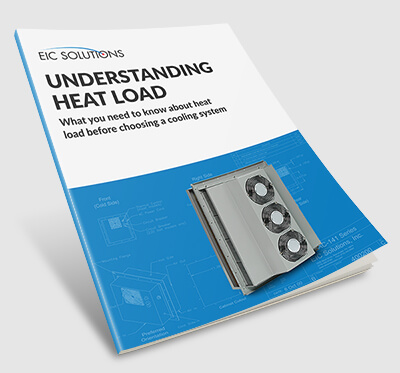# Guide to Heat Load | Thermoelectric Cooling## What is “Heat Load”? What factors contribute to it?

In this eBook we will cover:

• How to calculate external heat load
• How to calculate solar heat load
• How to calculate internal heat load